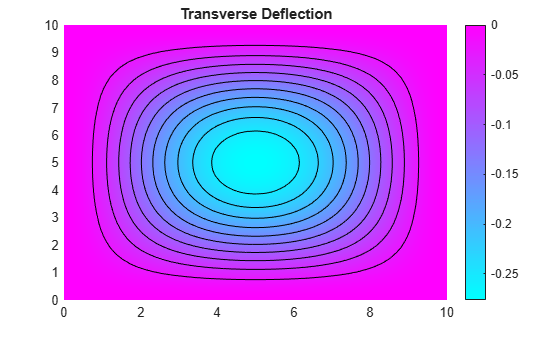# pdeCoefficientsToDouble

Convert symbolic PDE coefficients to `double` format

## Syntax

``coeffs = pdeCoefficientsToDouble(symCoeffs)``

## Description

example

````coeffs = pdeCoefficientsToDouble(symCoeffs)` converts the symbolic objects of the structure `symCoeffs` into double-precision numbers. The output is a structure `coeffs` that can then be used to define the coefficients of a PDE model by calling `specifyCoefficients` in Partial Differential Equation Toolbox™. The structure `coeffs` contains the coefficients `m`, `d`, `c`, `a`, and `f` for the PDE system with the form $m\frac{{\partial }^{2}u}{\partial {t}^{2}}+d\frac{\partial u}{\partial t}-\nabla ·\left(c\otimes \nabla u\right)+au=f$that can be solved in PDE Toolbox. For more information, see Equations You Can Solve Using PDE Toolbox (Partial Differential Equation Toolbox).```

## Examples

collapse all

Create a symbolic PDE that represents the Laplacian of the variable `u(x,y)`.

```syms u(x,y) f pdeeq = laplacian(u,[x y]) == f```
```pdeeq(x, y) =  ```

Extract the coefficients of the PDE as symbolic expressions and display their values.

```symCoeffs = pdeCoefficients(pdeeq,u,'Symbolic',true); structfun(@disp,symCoeffs)```
`$0$`
`$0$`
`$\left(\begin{array}{cc}-1& 0\\ 0& -1\end{array}\right)$`
`$f$`
`$0$`

`pdeCoefficients` converts the symbolic PDE into a scalar PDE equation of the form

$m\frac{{\partial }^{2}u}{\partial {t}^{2}}+d\frac{\partial u}{\partial t}-\nabla \cdot \left(c\nabla u\right)+au=f$,

and extract the coefficients `a`, `c`, `m`, `d`, and `f` into the structure `symCoeffs`.

Choose a value for `f`. Since `symCoeffs` are symbolic objects, use `pdeCoefficientsToDouble` to convert the coefficients to `double` data type. The coefficients with `double` data type are valid inputs for the `specifyCoefficients` function in the PDE Toolbox.

```symCoeffs = subs(symCoeffs,f,-3); coeffs = pdeCoefficientsToDouble(symCoeffs)```
```coeffs = struct with fields: a: 0 c: [4x1 double] m: 0 d: 0 f: -3 ```

Solve a system of two second-order PDEs. You can solve the PDE system by extracting the PDE coefficients symbolically using `pdeCoefficients`, converting the coefficients to double-precision numbers using `pdeCoefficientsToDouble`, and specifying the coefficients in the PDE model using `specifyCoefficients`.

The system of PDEs represents the deflection of a clamped structural plate under a uniform pressure load. The system of PDEs with the dependent variables ${u}_{1}$ and ${u}_{2}$ is given by

$-{\nabla }^{2}{u}_{1}+{u}_{2}=0$,

$-D{\nabla }^{2}{u}_{2}=p$,

where $D$ is the bending stiffness of the plate given by

$D=\frac{E{h}^{3}}{12\left(1-{\nu }^{2}\right)}$,

and $E$ is the modulus of elasticity, $\nu$ is Poisson's ratio, $h$ is the plate thickness, ${u}_{1}$ is the transverse deflection of the plate, and $p$ is the pressure load.

Create a PDE model for the system of two equations.

`model = createpde(2);`

Create a square geometry. Specify the side length of the square. Then include the geometry in the PDE model.

```len = 10.0; gdm = [3 4 0 len len 0 0 0 len len]'; g = decsg(gdm,'S1',('S1')'); geometryFromEdges(model,g);```

Specify the values of the physical parameters of the system. Let the external pressure $p$ be a symbolic variable `pres` that can take any value.

```E = 1.0e6; h_thick = 0.1; nu = 0.3; D = E*h_thick^3/(12*(1 - nu^2)); syms pres```

Declare the PDE system as a system symbolic equations. Extract the coefficients of the PDE and return them in symbolic form.

```syms u1(x,y) u2(x,y) pdeeq = [-laplacian(u1) + u2; -D*laplacian(u2) - pres]; symCoeffs = pdeCoefficients(pdeeq,[u1 u2],'Symbolic',true)```
```symCoeffs = struct with fields: m: 0 a: [2x2 sym] c: [4x4 sym] f: [2x1 sym] d: 0 ```

Display the coefficients `m`, `a`, `c`, `f`, and `d`.

`structfun(@disp,symCoeffs)`
`$0$`
`$\left(\begin{array}{cc}0& 1\\ 0& 0\end{array}\right)$`
`$\left(\begin{array}{cccc}1& 0& 0& 0\\ 0& 1& 0& 0\\ 0& 0& \frac{25000}{273}& 0\\ 0& 0& 0& \frac{25000}{273}\end{array}\right)$`
`$\left(\begin{array}{c}0\\ \mathrm{pres}\end{array}\right)$`
`$0$`

Substitute a value for `pres` using the `subs` function. Since the outputs of `subs` are symbolic objects, use the `pdeCoefficientsToDouble` function to convert the coefficients to the `double` data type, which makes them valid inputs for the PDE Toolbox.

```symCoeffs = subs(symCoeffs,pres,2); coeffs = pdeCoefficientsToDouble(symCoeffs)```
```coeffs = struct with fields: a: [4x1 double] c: [16x1 double] m: 0 d: 0 f: [2x1 double] ```

Specify the PDE coefficients for the PDE model.

```specifyCoefficients(model,'m',coeffs.m,'d',coeffs.d, ... 'c',coeffs.c,'a',coeffs.a,'f',coeffs.f);```

Specify spring stiffness. Specify boundary conditions by defining distributed springs on all four edges.

```k = 1e7; bOuter = applyBoundaryCondition(model,'neumann','Edge',(1:4), ... 'g',[0 0],'q',[0 0; k 0]);```

Specify the mesh size of the geometry and generate a mesh for the PDE model.

```hmax = len/20; generateMesh(model,'Hmax',hmax);```

Solve the model.

`res = solvepde(model);`

Access the solution at the nodal locations.

`u = res.NodalSolution;`

Plot the transverse deflection of the plate.

```numNodes = size(model.Mesh.Nodes,2); figure; pdeplot(model,'XYData',u(1:numNodes),'contour','on') title 'Transverse Deflection'```Find the transverse deflection at the plate center.

`wMax = min(u(1:numNodes,1))`
```wMax = -0.2763 ```

Compare the result with the deflection at the plate center computed analytically.

```pres = 2; wMax = -.0138*pres*len^4/(E*h_thick^3)```
```wMax = -0.2760 ```

## Input Arguments

collapse all

Coefficients of PDE in symbolic form, specified as a structure of symbolic expressions.

## Output Arguments

collapse all

Coefficients of PDE in double precision, returned as a structure of double-precision numbers.

Introduced in R2021a

## Support

#### Mathematical Modeling with Symbolic Math Toolbox

Get examples and videos